## Similar Triangles

Let’s begin our discussion of similar triangles with an example.

### Guided Example

Mary was out in the yard one day and had her two daughters with her. She was doing some renovations and wanted to know how tall the house was. She noticed a shadow 3 feet long when her daughter was standing 12 feet from the house and used it to set up figure 1.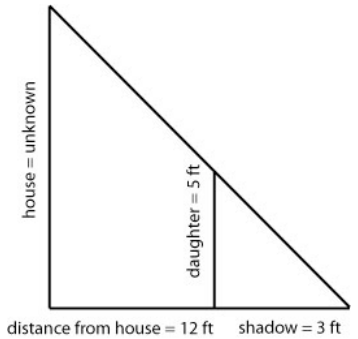Figure 1.

We can take that drawing and separate the two triangles as follows allowing us to focus on the numbers and the shapes.

These triangles are what are called similar triangles. They have the same angles and sides in proportion to each other. We can use that information to determine the height of the house as seen in figure 2.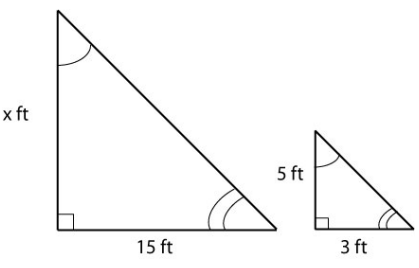Figure 2.

To determine the height of the house, we set up the following proportion:

$\displaystyle\frac{x}{15}=\frac{5}{3}\\$

Then, we solve for the unknown x by using cross products as we have done before:

$\displaystyle{x}=\frac{5\times{15}}{3}=\frac{75}{3}=25\\$

Therefore, we can conclude that the house is 25 feet high.

### Example 1

Use the Similar Triangles process to determine the length of the missing side. Set up the proportions in as many ways as possible and show the results are all the same.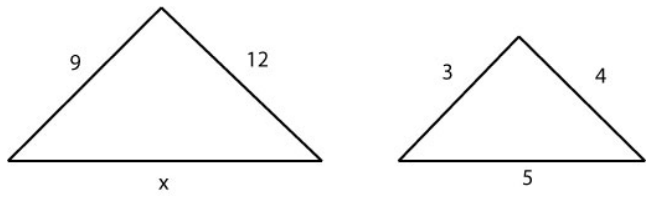15 units

### Example 2

Use the similar triangles process to determine the length of the missing sides. You may need to redraw your triangles to set up the proportions correctly.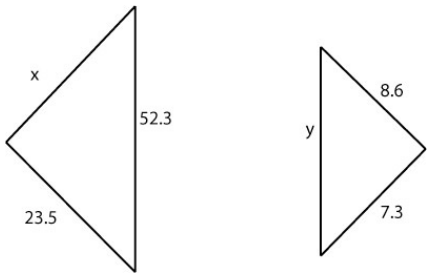#### Solution

x = 27.7 (rounded), y = 16.2 (rounded)

### Example 3

Given the similar triangles below, find the missing lengths. Round to tenths as needed. Feel free to redraw the triangles so you can see the proportional sides.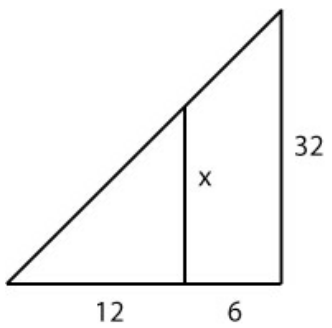21.3 (rounded)

### Example 4

#### Applications of Similar Triangles

Mary (from the application that started this topic), decides to use what she knows about the height of the roof to measure the height of her second daughter. If her second daughter casts a shadow that is 1.5 feet long when she is 13.5 feet from the house, what is the height of the second daughter? Draw an accurate diagram and use similar triangles to solve.

2.5 ft

## Square Roots

Before we get to our last topic in this lesson—the Pythagorean Theorem,—we need to know a little bit about square roots.

• The square root of a number is that number which, when multiplied times itself, gives the original number. On your calculator, look for $\sqrt\text{ }\\$ to compute square roots. $\sqrt{16}=4\\$ because 4 × 4 = 16
• A perfect square is a number whose square root is a whole number. The square root of a non-perfect square is a decimal value. $\sqrt{16}\\$ is a perfect square.$\sqrt{19}\\$ is not a perfect square.
• To obtain a decimal value for non-perfect square roots on your calculator, you may need to change the settings under your MODE button. Check your owner’s manual for help if needed. $\sqrt{19}=4.36\\$ (rounded to hundredths)

### Example 5

Find the square root of each of the following. Round to two decimal places if needed. Indicate those that are perfect squares and explain why.

1. $\sqrt{169}\\$
2. $\sqrt{31}\\$
3. $\sqrt{9}\\$

#### Solutions

1. 13 (perfect square)
2. 5.57 (not a perfect square)
3. 9 (perfect square)

### Example 6

Find the square root of each of the following. Round to two decimal places if needed. Indicate those that are perfect squares and explain why.

1. $\sqrt{225}\\$
2. $\sqrt{17}\\$
3. $\sqrt{324}\\$

#### Solutions

1. 15 (perfect square)
2. 4.12 (not a perfect square)
3. 18 (perfect square)

## The Pythagorean Theorem

The mathematician Pythagoras proved the Pythagorean theorem. The theorem states that given any right triangle with sides a, b, and c as below, the following relationship is always true: a2 + b2 = c2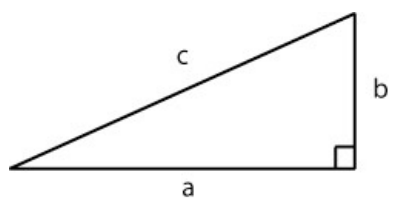a2 + b2 = c2

• The triangle must be a right triangle (contains a 90º angle).
• The side c is called the hypotenuse and always sits across from the right angle.
• The lengths a and b are interchangeable in the theorem, but c cannot be interchanged with a or b. In other words, the location of c is very important and cannot be changed.

### Example 7

Use the Pythagorean theorem to find the missing sides length for the triangle given below. Round to the tenths place.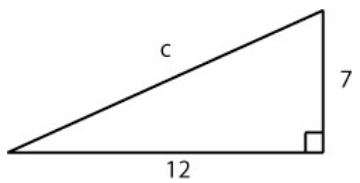13.9 (rounded)

### Example 8

Use the Pythagorean theorem to find the missing sides length for the triangle given below. Round to the tenths place.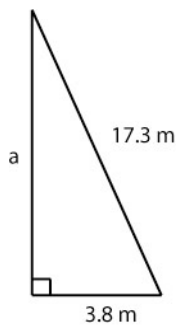16.9 m (rounded)

### Example 9

Use the Pythagorean theorem to find the missing sides length for the triangle given below. Round to hundredths.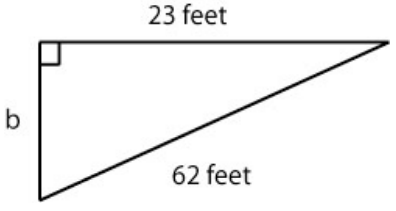#### Solution

57.58 ft (rounded)

### Example 10

#### Applications of the Pythagorean Theorem

In NBA Basketball, the width of the free-throw line is 12 feet. A player stands at one exact corner of the free throw line (Player 1) and wants to throw a pass to his open teammate across the lane and close to the basket (Player 2). If his other teammate (Player 3—heavily guarded) is directly down the lane from him 16 feet, how far is his pass to the open teammate? Fill in the diagram below and use it to help you solve the problem.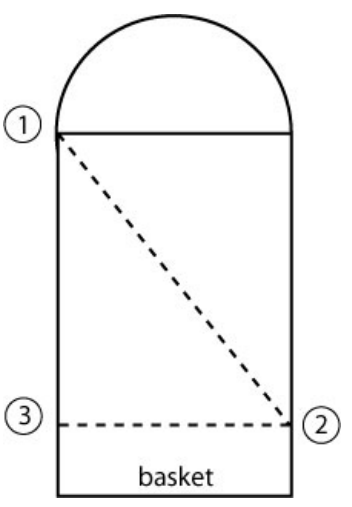20 ft